• ## 计算数组元素之和

千次阅读 2019-08-04 17:22:45
#include <stdio.h> int main() { int array = {1, 2, 3, 4, 5, 6, 7, 8, 9, 0}; int sum, loop; sum = 0; for(loop = 9; loop >= 0; loop--){ sum = sum + array[loop]; } ......
#include <stdio.h>
int main() { int array = {1, 2, 3, 4, 5, 6, 7, 8, 9, 0}; int sum, loop;
sum = 0;

for(loop = 9; loop >= 0; loop--){
sum = sum + array[loop];
}

printf("元素和为：%d",sum);

return 0;

}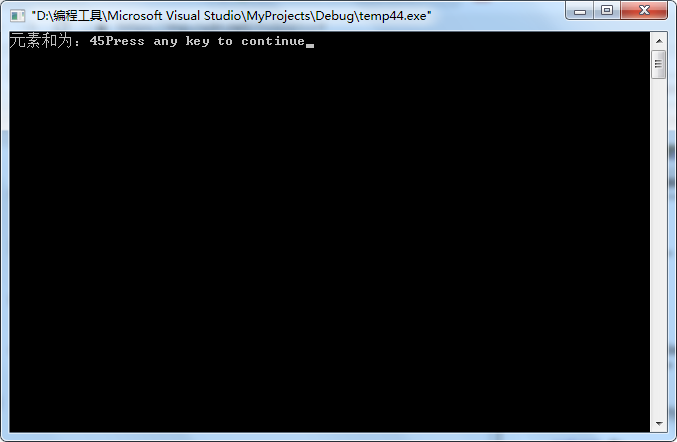展开全文C
• HashMap如何计算数组下标 首先我们看看String的hashCode是如何计算的（出自JDK1.8.0 211 java.lang.String 1452行—1476行） /** * Returns a hash code for this string. The hash code for a * {@code String} ...
讨论 代码环境为JDK1.8.0 211
HashMap如何计算数组下标
首先我们看看String的hashCode是如何计算的（出自JDK1.8.0 211 java.lang.String 1452行—1476行）
/**
* Returns a hash code for this string. The hash code for a
* {@code String} object is computed as
* <blockquote><pre>
* s*31^(n-1) + s*31^(n-2) + ... + s[n-1]
* </pre></blockquote>
* using {@code int} arithmetic, where {@code s[i]} is the
* <i>i</i>th character of the string, {@code n} is the length of
* the string, and {@code ^} indicates exponentiation.
* (The hash value of the empty string is zero.)
*
* @return  a hash code value for this object.
*/
public int hashCode() {
int h = hash;
if (h == 0 && value.length > 0) {
char val[] = value;

for (int i = 0; i < value.length; i++) {
h = 31 * h + val[i];
}
hash = h;
}
return h;
}

String类重写了hashCode()函数，计算字符串的hashCode的公式为：

s

[

0

]

∗

3

1

n

−

1

+

s

[

1

]

∗

3

1

n

−

2

+

.

.

.

+

s

[

n

−

1

]

s*31^{n-1}+s*31^{n-2}+...+s[n-1]

我们来举个例子：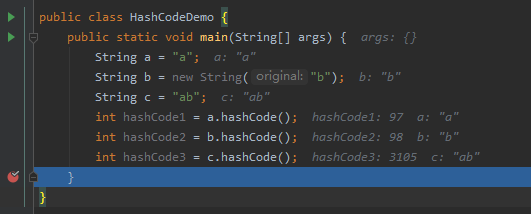经过debug我们得知三个字符串的hashCode()返回值，我们来通过计算公式模拟一下： 字符串“a”，长度n=1，字符‘a’=97，代入公式得：

s

[

0

]

∗

3

1

n

−

1

+

s

[

1

]

∗

3

1

n

−

2

+

.

.

.

+

s

[

n

−

1

]

=

97

∗

3

1

1

−

1

=

97

s*31^{n-1}+s*31^{n-2}+...+s[n-1]=97*31^{1-1}=97

字符串“ab”，长度n=2，字符‘a’=97，‘b’=98，代入公式得：

s

[

0

]

∗

3

1

n

−

1

+

s

[

1

]

∗

3

1

n

−

2

+

.

.

.

+

s

[

n

−

1

]

=

97

∗

3

1

2

−

1

+

98

∗

3

1

1

−

1

=

3105

s*31^{n-1}+s*31^{n-2}+...+s[n-1]=97*31^{2-1}+98*31^{1-1}=3105

好了知道了如何计算String的hashCode，再来看看HashMap的hash()函数是如何利用hashCode的：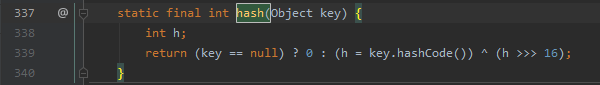如果key对象为null的话，就返回一个0，当然String对象为null的话，强行计算hashCode()是会抛出空指针异常的。如果key对象不是为null，那么获取key的hashCode，然后与右移位16位后的hashCode进行异或操作（关于异或操作，总结：不同，结果为1，相同，结果为0），异或的结果就位hash()函数返回值。我们以String的hashCode()为例，图解计算一次hash()返回值：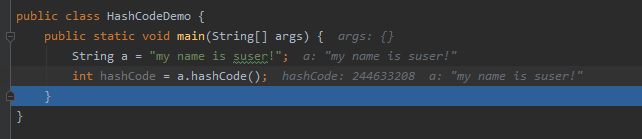通过debug，我们知道“my name is suser！”的hashCode返回值为：244633208，然后通过进制转换为二进制，方便计算我们图解一下计算过程：得到hash(key)=244629740后，下一步计算数组下标，我们看源码中第630行：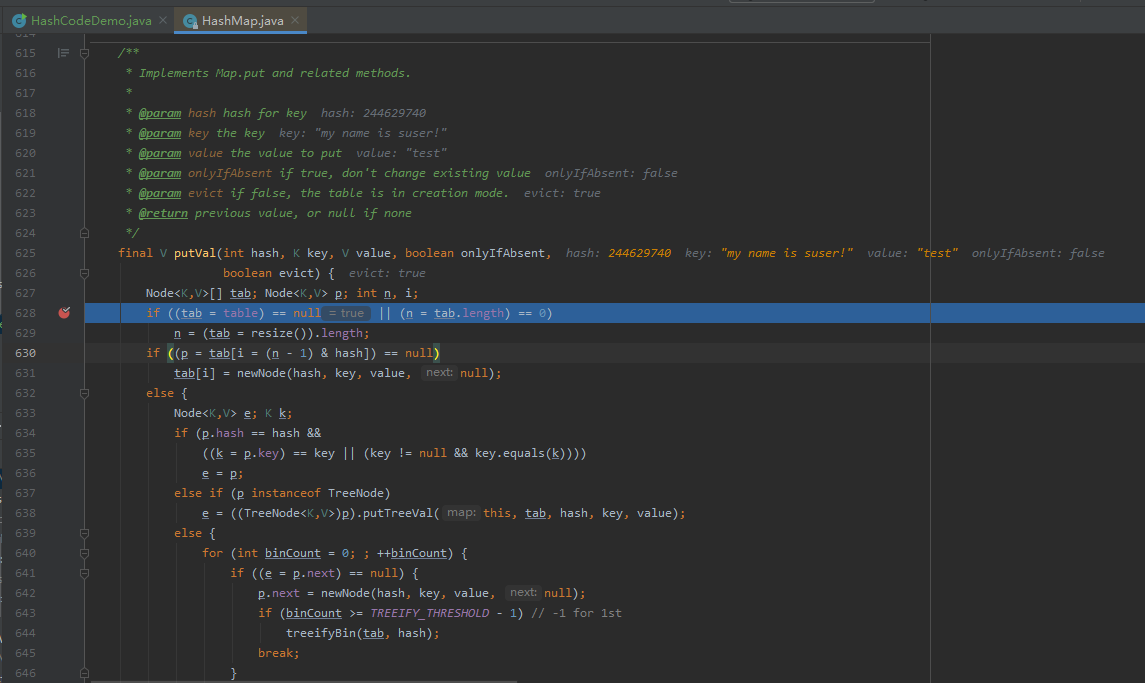tab[i = (n - 1) & hash]

在JDK1.8中，HashMap底层为数组+链表或红黑树 i是什么？i就是数组下标； n是什么？n是数组长度，默认为16 hash就是hash(key)函数的返回值，244629740为例，这里要得出下标，需要进行一次&（与运算）（同为1，则1，不然则为0） 这里，假设HashMap刚刚创建，也没有经过扩容操作，数组长度这时为默认的16，来算算下标：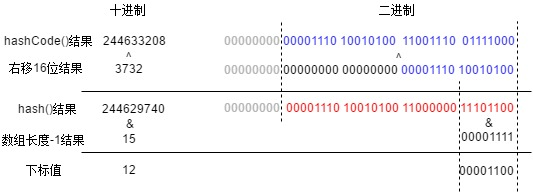最终计算得下标值为12。 下面，通过debug结果，给出证据，证明其下标值确定为12：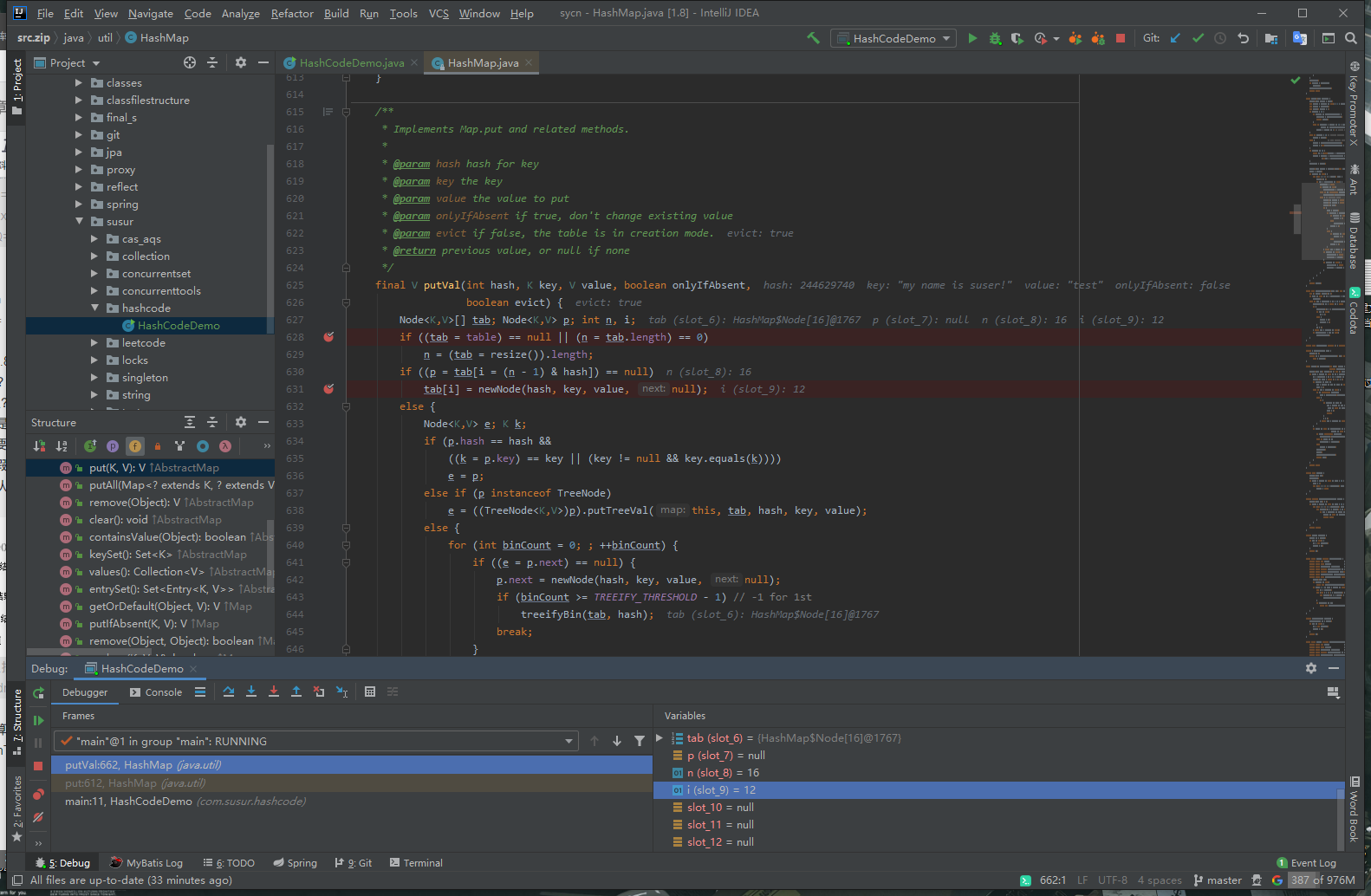625行debug信息显示hash(key)返回值，key值等关键信息 630行debug信息显示此时HashMap数组长度为默认16，还未经过扩容操作 631行debug信息显示i的值为12，与我推想的结果一致

总结一下： HashMap中数组下标值的计算过程，大致分为如下几步：获取key.hashCode()，然后将hashCode高16位和低16位异或（^）操作，然后与当前数组长度-1结果进行与（&）操作，最终结果就是数组的下标值。 至于由于当前结点（键值对）的加入，导致当前HashMap中容量超过了阈值而扩容2倍，扩容后导致每个结点重新计算下标值（称为新下标值），新下标值只有两种可能：一、key的hash()返回值新加入与（&）操作计算的一位为0，则下标值与原下标值相同。二、key的hash()返回值新加入与（&）操作计算的一位为1，则计算出来的下标值=原下标值+原数组长度。 如果key对象为Integer，他的hashCode()直接返回的value。

展开全文java
• 利用C语言可以实现对数组的各种操作，如输入数组元素，输出数组元素、求数组元素平均值、输出数组元素最大值、输出数组元素最小值、查找某数值元素是否存在、给数组元素排序等功能。本压缩文件中是上述功能对应的...
• 数组 1.数组的创建： 元素类型 数组名 [常量或者常量表达式] 如：int arr1; 注：即使是被const修饰的变量也不能作为[]中的内容，它本质上依然属于变量，只是具有常量属性 2.数组的初始化：  创建数组时...
数组

1.数组的创建：
元素类型 数组名 [常量或者常量表达式]  如：int arr1; 注：即使是被const修饰的变量也不能作为[]中的内容，它本质上依然属于变量，只是具有常量属性

2.数组的初始化：
创建数组时，若不指定数组大小一定要初始化，但是初始化后可以不指定数组大小
注：（1）字符串放在数组里时，默认最后还有\0,字符数组则不是，有啥放啥
（2）char *p="abcdef",p中放的是'a'的地址，通过这个地址可以找到a,以及a后面的字符
3.下标引用操作符: []    数组通过下标（从0开始）来访问，数组大小计算：len=sizeof(arr1)/sizeof(arr1)

4.一维数组在内存中连续存储：用语句printf("&arr1[%d]=%p\n",i,&arr1[i]);来查看各个元素地址

5.指针
（1）32位平台上指针大小为4个字节，64位则是8个字节
（2）指针是一个用来存放地址的一个变量
（3）对于一维数组，除了sizeof(单个数组名)、&单个数组名以外，其它地方出现的都是代表数组首元素地址
（4）获得数组中每个元素地址：A.数组名+整数（首元素地址+整数） B.&arr1[i]   （5）int *p=arr1，arr1+i等价于 p+i,因为p里保存的就是首元素地址arr1,*(p+i)就是元素本身，p[i]也是元素本身，因为p相当于shuzumrr1

6.二维数组的创建和初始化
（1）下标第一项可以省，第二项不能省
（2）也是通过下标来访问
（3）二维数组在内存中也是连续存储的，（只是一行完了再一行）
（4）二维数组arr1,看成一维数组是三个元素，arr1、arr1、arr1，只是这三个元素又是一个数组而已
（5）二维数组arr1,其中单独的数组名arr1代表的是将其看为一维数组时第一个元素arr1的地址，而仅仅是这个二位数组的首地址应该是&arr1. （6）二维数组指针访问更加方便（初始化直接利用指针往后算，输出时还有利用两个for循环有序输出）

7.关于数组的运算
注意：sizeof()求得是变量或者空间开辟空间的大小，而strlen()函数参数接收的必须是一个有效地址
（1）

#include <stdio.h>
int main()
{
int a[]={1,2,3,4};
printf("%d\n",sizeof(a));  //16 整个数组大小
printf("%d\n",sizeof(a+0));  //4 首元素地址
printf("%d\n",sizeof(*a));  //4 首元素所占空间大小，1个整型
printf("%d\n",sizeof(a+1));  //4 第二个元素地址大小
printf("%d\n",sizeof(a));  //4 第二个元素所占空间大小
printf("%d\n",sizeof(&a));  //4 整个数组地址
printf("%d\n",sizeof(&a+1));  //4 跳到下一个地址开头，但是目前还是4
printf("%d\n",sizeof(&a));  //4 首元素地址
printf("%d\n",sizeof(&a+1));  //4 第二个元素的地址
return 0;
}
（2）

#include <stdio.h>
int main()
{
int a[]={1,2,3,4};
printf("%d\n",sizeof(a));  //16 整个数组大小
printf("%d\n",sizeof(a+0));  //4 首元素地址
printf("%d\n",sizeof(*a));  //4 首元素所占空间大小，1个整型
printf("%d\n",sizeof(a+1));  //4 第二个元素地址大小
printf("%d\n",sizeof(a));  //4 第二个元素所占空间大小
printf("%d\n",sizeof(&a));  //4 整个数组地址
printf("%d\n",sizeof(&a+1));  //4 跳到下一个地址开头，但是目前还是4
printf("%d\n",sizeof(&a));  //4 首元素地址
printf("%d\n",sizeof(&a+1));  //4 第二个元素的地址
return 0;
}
注意：只要是地址，不管是整型数组，还是字符数组，只要是32位平台，都是4个字节

（3）

#include <stdio.h>
int main ()
{
char arr[]={'a','b','c','d','e','f'};
printf("%d\n",strlen(arr));  //19随机值，不知道什么时候会遇到\0
printf("%d\n",strlen(arr+0));  //19随机值
//printf("%d\n",strlen(*arr));  //系统奔溃，字符a不是一个有效地址
//printf("%d\n",strlen(arr));  //系统奔溃，字符a不是一个有效地址
printf("%d\n",strlen(&arr));  //19随机值 先找到整个数组地址，然后从第一个字符往后找，所以和1、2随机数字是一样
printf("%d\n",strlen(&arr+1));  //13随机值,这里因为整个数组地址加1，相当于把它跳过去了，19-6=13（注意）
printf("%d\n",strlen(&arr+1));  // 18随机值，这里因为是从第二个元素地址开始往后找，19-1=18（注意）
return 0;
}

（4）

#include <stdio.h>
int main()
{
char *p="abcdef";
printf("%d\n",sizeof(p));  //4 ，p存放首元素a的地址，指针大小都为4
printf("%d\n",sizeof(p+1));  //4，元素b的地址
printf("%d\n",sizeof(*p));  //1，首元素所占空间大小
printf("%d\n",sizeof(p));  //1，首元素所占空间大小
printf("%d\n",sizeof(&p));  //4，给指针变量取地址，还是个地址，所以4个字节
printf("%d\n",sizeof(&p+1));  //4，p指向下一个元素的地址（指向b的地址）
printf("%d\n",sizeof(&p+1));  //4 第二个元素的地址
return 0;
}
注意：&和*的区分使用啦

（5）

#include <stdio.h>
int main()
{
char *p="abcdef";
printf("%d\n",strlen(p)); //6，p存的是首字符a的地址，从这个入口进去，直到遇到\0结束，（\0不算在内）
printf("%d\n",strlen(p+1));  //5，p+1指向第二个字符，再与第一个一样
printf("%d\n",strlen(*p)); //系统奔溃，字符a不算一个有效地址
printf("%d\n",strlen(p));  //系统奔溃，字符a不算一个有效地址
printf("%d\n",strlen(&p)); //随机值,(注意：易错，这里给指针变量取地址还是地址，但是从这个地址开始查找，后面情况就不确定了)
printf("%d\n",strlen(&p+1));  //随机值（问题和上一个一样，只是p指向的元素向后挪了一个）
printf("%d\n",strlen(&p+1)); //5，入口地址从第二个元素开始
return 0;
}

（6）

#include <stdio.h>
int main()
{
int a={0};
printf("%d\n",sizeof(a));  //48,该数组共有12个元素，每个元素4字节，一个48字节
printf("%d\n",sizeof(a));   //4，就代表第一个元素所占空间大小
printf("%d\n",sizeof(a));  //16 将二维数组看成一维数组，则该数组共有3个元素，a为第一个元素，（即第一行）
printf("%d\n",sizeof(a+1));  //4 a看成一维数组的数组名，代表首地址，再加一，第二个元素地址
printf("%d\n",sizeof(a+1));  //4，a是首元素地址（第一行），加一跳过第一行来到第二行首元素位置(注意：算地址都是4位)
printf("%d\n",sizeof(&a+1)); //4  a是一维数组的数组名，对它取地址代表整个数组，再加一，来到第二行（注意：同上一个）
printf("%d\n",sizeof(*a));  //16 ，a是首元素地址（第一行地址），对其解引用，为第一行
printf("%d\n",sizeof(a));  //16 虽然不存在，但是和a一样，有4个元素，每个元素4字节，工16字节
return 0;
}
注意：地址加整数算的还是地址


展开全文c语言
• 数组元素的总和是指数组中所有元素（或数字）的总和。数组元素可以是整数（int）或十进制数字（float或double）。 方法1：使用for循环 这是一种传统且最常用的方法，其中使用for循环来迭代数组。 在每次迭代中，...
数组元素的总和是指数组中所有元素（或数字）的总和。数组元素可以是整数（int）或十进制数字（float或double）。

方法1：使用for循环 这是一种传统且最常用的方法，其中使用for循环来迭代数组。 在每次迭代中，将当前数组元素添加到一个保存数组元素总和的变量中。 在循环开始之前，此变量被初始化为0。例如，
​
public class ArraySumCalculator {
public static void main(String[] args) {
int[] array = { 1, 34, 67, 23, -2, 18 };
// variable to hold sum of array elements
int sum = 0;
// iterate using a for loop
for (int loopCounter = 0; loopCounter < array.length; loopCounter++) {
// get current array element
int element = array[loopCounter];
sum += element;
}
System.out.println("Sum of array elements is: " + sum);
}
}

​
sum += element 是的简写 sum = sum + element
上面的程序打印

数组元素的总和是：141

for该程序中的for-each循环也可以用如下所示的循环代替。
		for (int element : array) {
sum += element;
}

方法2：在Java 8使用Arrays.stream 此方法使用流概念在Java 8.呼叫引入stream上方法java.util.Arrays类供给它的阵列作为参数。此方法返回数组元素流。在此流上 调用sum方法将提供数组中元素的总和。例如，
import java.util.Arrays;
import java.util.stream.IntStream;

public class ArraySumCalculator3 {

public static void main(String[] args) {
int[] array = { 1, 34, 67, 23, -2, 18 };
// get stream of elements
IntStream stream = Arrays.stream(array);
// add the elements in stream
int sum = stream.sum();
System.out.println("Sum of array elements is: " + sum);
}
}

请注意，调用stream方法返回a java.util.stream.IntStream，它是整数值的流。这是因为提供给stream方法的数组是整数数组。 如果数组的类型为double，则流将为java.util.stream.DoubleStream 上面的代码，也可以将其简化为以下一行。
int sum = Arrays.stream(array).sum();
该程序的输出与上一个相同。

方法3：在Java 8中使用IntStream Java 8引入了Java 8java.util.stream.IntStream，这是一个元素序列。它有一个of以数组为参数的方法，并返回数组元素的流。返回的流上的 调用sum方法提供数组元素的总和。例如，
import java.util.stream.IntStream;

public class ArraySumCalculator5 {

public static void main(String[] args) {
int[] array = { 1, 34, 67, 23, -2, 18 };
// get stream of elements
IntStream stream = IntStream.of(array);
// add the elements in stream
int sum = stream.sum();
System.out.println("Sum of array elements is: " + sum);
}
}
上面的代码可以简化为如下所示的单行代码。
int sum = IntStream.of(array).sum();

甲 减少 操作通过组合操作的反复应用，例如找到一组数字的总和或最大值，或累积的元素到一个列表中需要输入元件和将它们组合的一个序列到单个汇总结果。

java.util.stream.IntStream有一个带有reduce2个参数的方法。首先是所需操作的标识元素。对于加法运算，标识为0。 第二个参数是类型的参数，java.util.function.InitBinaryOperator它是一个函数接口，该函数接口具有applyAsInt将运算应用于所提供的操作数的方法。 可以使用Lambda表达式提供对此方法的实现。例子，
import java.util.stream.IntStream;

public class ArraySumCalculator7 {

public static void main(String[] args) {
int[] array = { 1, 34, 67, 23, -2, 18 };
// get stream of elements
IntStream stream = IntStream.of(array);
// add the elements in stream using reduction
int sum = stream.reduce(0, (element1, element2) -> element1 + element2);
System.out.println("Sum of array elements is: " + sum);
}
}
注意reduce方法的参数。第一个是0，这是要添加的标识。第二个是lambda表达式，它表示“对提供的元素应用加法运算”。 此方法将遍历所有数组元素并执行这些元素的添加。 以上方法可以简化为单线。
int sum = IntStream.of(array).reduce(0, (element1, element2) -> element1 + element2);

展开全文• js对象数组计算总计Knowing how to quickly iterate through an array and count objects is deceptively simple. The length() method will tell you the total number of values in the array, but what if you ...java python javascript js vue
• C语言中，定义数组之后可以通过sizeof(a)/sizeof(a)的方法获得数组长度。 然而，当数组当作参数到子函数中，以上获取数组长度得到的结果永远是1. 因为，a是函数参数，到了本函数中，a只是一个指针（地址，系统...C
• 递归计算数组元素之和 package abc; public class Test {  static int[] a = {4,6,7,43,3,9,89}; // 测试数组  static int sum = 0; // 定义一个变量用于放入数组元素的和  static int num = a.length;...JAVA 程序 递归
• ## 计算数组的相似度

千次阅读 2018-11-20 21:54:39
Problem G: 计算数组的相似度 Time Limit: 1 Sec Memory Limit: 128 MB Submit: 1955 Solved: 584 [Submit][Status][Web Board] Description Jaccard系数主要用于计算个体间的相似度。对于两个集合A、B，定义其...
• //ES6 数组reduce()方法 console.log('reduce:'+arr.reduce((a,b)=>a+b)); //ES6 forEach()方法.该方法内的返回值会对原数组进行操作 var total = 0; arr.forEach(function(a){ total += a; }); console.lo...js
• public class ArrayDemo03 { public static void main(String[] args) { ...//计算数组的和 int sum=0; for (int i = 0; i < a.length; i++) { sum+=a[i]; } System.out.println("sum="+sum);java
• a=1.2 a=1.3 i=0 for var in ${a[@]} do let i++ sum=echo$sum $var | awk '{ print$1+$2 } '` done echo$sum $i | awk ' { printf("%.3f",$1/$2) } ' 转载于:ht... • strlen 是一个函数，它用来计算指定字符串 str 的长度，但不包括结束字符（sizeof()包括结束字符） #include<stdio.h> #include<string.h> int main(){ char a[]={"abcdd"}; printf("用strlen... • #include<stdio.h>... int a; int max,row,col,i,j,m,n; row = col =1; printf("Input m, n:"); scanf("%d,%d",&m,&n); printf("Input %d*%d array:\n",m,n); for(i=0;i<mc语言 • #include "stdio.h"#include "windows.h"int a[]={1,2,3,4,5,6,7,8,9,10,11,12,13,14,15};... return a[n-1]+sum(a,n-1);}main(){ int i; system("cls"); printf("\n the ar... • c语言中，定义数组后可以用sizeof命令获得数组的长度（可容纳元素个数）。 例如： int data; int length; length=sizeof(data)/sizeof(data); //数组占内存总空间，除以单个元素占内存空间大小 printf("length... • 计算数组长度时可以用 int array[] = {1, 2, 3}; sizeof(array) = 12; int length=sizeof(array)/sizeof(array)=3; char array[] = “abc”; sizeof(array) = 4;//考虑’\0’ strlen(array)=3//不考虑’\0’ int ...c语言 • sizeof计算对象所占内存的大小,判断操作数的类型长度,以字节为单位； 指针在32位系统占4个字节，在64位系统占8个字节，本实例都在32位机器上操作。 sizeof 求大小看的仅仅是它的类型大小，而非其他。所以我们在计算... • 今天碰到一个小问题，因为这个sizeof只适用于数组名，如果不小心换成了指向数组的指针，那么很不幸，sizeof(pointer) 等于4，那就悲剧了。 static const CodecProfile codec_profile[] = { { .codec_id = AV_CODEC... • 文章转载自...1、计算特定值的重复个数 let counts = (arr, value) => arr.reduce((a, v) => v === value ? a + 1 : a + 0, 0); console.log('数组counts中"李"出现的次数是：...javascript js • 按照传统，先给结论：sizeof(数组名) 的值是这个数组所占据的内存的大小，单位是字节(bytes)，在32位机器上，假设一个int型的数组a，里面一共有10个int类型元素，那么sizeof(a)的值就是10 * 4 = 40 原文链接：...c sizeof • ## sizeof是如何计算数组大小的 万次阅读 多人点赞 2018-01-23 16:36:17 sizeof是如何计算数组大小的 char *ptr; char arr; sizeof(ptr) =4; sizeof(arr) =10;请问是如何计算数组大小的？这里只是把地址传给sizeof啊 严格讲， sizeof(arr) =10; 这里只是把地址传给...sizeof • C#计算数组的最大值，最小值，总和，平均数，众数 本人大学生C#小白一个，这是一个小的上机实验，发出来让各位大佬评价评价，觉得好的请点个赞或者收藏一下，不好请在评论区喷我就可以了。谢谢！ 代码如下 using ...C# • 有一个数组（或字符串）$arr=[‘a’,’b’,’c’,’a’,’d’,’z’,’y’]，由单个字符组成，求出现次数最多的字符出现的次数PHP 算法
• 从键盘输入10个整型数据，放入数组a 中，求其最大值，并输出。 **要求输入提示信息为：无输入提示信息 **要求输入格式为："%d" **要求输出格式为："max=%d\n" #include <stdio.h> int main() { int i; ...
• ## 数组偏移量计算

千次阅读 2019-07-26 18:34:39
数组偏移量计算一维数组二维数组三维数组 一维数组 A 求A 偏移量为 2 即为下标 二维数组 设有数组A[1…5] [0…3] 求偏移量A  [ 2] 已知 m = 5，n = 3， i = 2 ，j = 2 行为主序 d = i * n + （j - 1...
• C++实现计算数组逆序对数目 用到的工具函数 计算数组的长度 template&lt;class T&gt; int length(T &amp;arr) { return sizeof(arr) / sizeof(arr); } 计算逆序对函数 void count...C++ 逆序对 归并排序 算法
• 通过指针变量输出整型数组a的10个元素。解：程序：#includeint main(){ int i, a, *p=a; printf("please enter 10 integer numbers:"); for (i = 0; i { scanf("%d", p++); } p = a; for (i = 0; i { printf...
• ## 数组长度计算

万次阅读 2018-07-30 22:46:25
可以用来计算数组的大小（数组所占字节数），但不是长度。 不过可以用它除以数组单个元素所占字节数计算长度： a = {1,2,3,4,5,,6,7}; length = sizeof(a)/sizeof(a); C++中可以用上述方法，不过还可以......